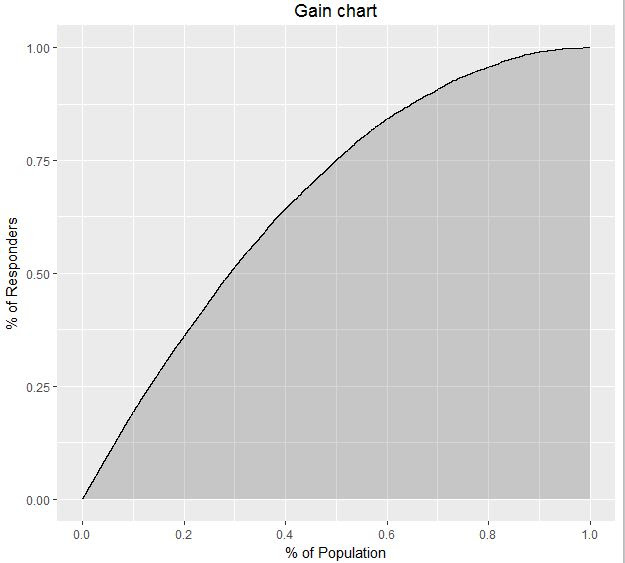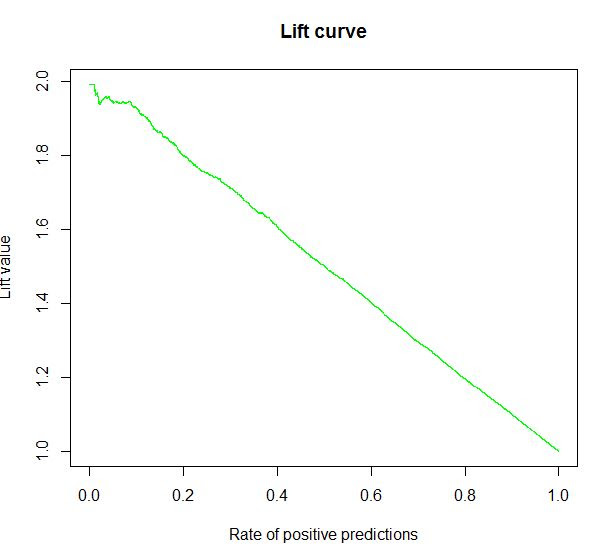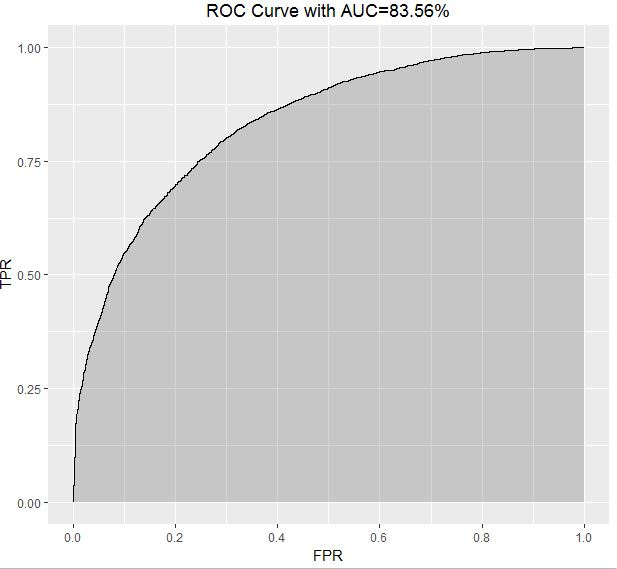### Long H. Nguyen

Knowledge worths sharing!

Email

# Metrics for model evaluation

There are a few popular metrics for model evaluation:

• Gain chart: = Gain at a given decile level is the ratio of cumulative number of targets (events) up to that decile to the total number of targets (events) in the entire data set.
• Lift chart: Lift is the ratio of gain % to the random expectation % at a given decile level.
• KS chart: K-S is a measure of the degree of separation between the positive and negative distributions. It is a very popular metrics in credit risk modeling.
• AUC plot: The ROC curve is created by plotting the true positive rate (TPR) against the false positive rate (FPR) at various threshold settings. This plot visualizes overall perfomance of models and is very useful metric for imbalance data.

This post will provide R code for plotting the charts using ggplot2 and computing these metrics so that you can reuse it easily.

## Library

``````library(ROCR)
library(ggplot2)
set.seed(1)
n=10000
# Randomly create score and ground truth for examples
score <- runif(n)
y <- (runif(n) < score)
``````

## Gain chart

``````gain.chart <- function(score, y) {
## Shuffle predicition
set.seed(0)
rand <- sample(1:length(y))
score <- score[rand]
y <- y[rand]

pred <- prediction(score, y)
perf <- performance(pred, measure = "tpr", x.measure = "rpp")
plot.data <- data.frame(xvals=unlist(perf@x.values), yvals=unlist(perf@y.values))
theme_update(plot.title = element_text(hjust = 0.5))
print(ggplot(plot.data, aes(x=xvals, ymin=0, ymax=yvals)) +
geom_ribbon(alpha=0.2) + geom_line(aes(y=yvals)) + scale_x_continuous(breaks = seq(0, 1, by = 0.2)) +
ggtitle("Gain chart") + xlab('% of Population') + ylab('% of Responders'))
results.sortedByProb<-order(score,decreasing=TRUE)
test_y.sorted <-y[results.sortedByProb]
for(k in 1:10){
cat('Gain ratio at ',k*10,'% is: ',round(sum(100*test_y.sorted[1:(length(test_y.sorted)*k/10)])/sum(test_y.sorted),digits=2),'% \n')
}
for(k in 1:10){
cat('# Responders at ',k*10,'% is: ',round(sum(test_y.sorted[(length(y)*(k-1)/10+1):(length(y)*k/10)])),'\n')
}
}
``````## Lift chart

``````lift.chart <- function(score, y) {
pred <- prediction(score, y)
perf <- performance(pred, measure = "lift", x.measure = "rpp")
plot(perf, main="Lift curve", col = 'green')
}
``````## KS

``````KS <- function(score, y){
perf <- performance(prediction(score, y), "tpr", "fpr")
ks <- max(attr(perf, "y.values")[] - (attr(perf, "x.values")[]))
return(ks)
}
``````

## AUC chart

``````roc.chart <- function(score, y) {
pred <- prediction(score, y)
perf <- performance(pred, measure = "tpr", x.measure = "fpr")
auc <- performance(pred, measure = "auc")
auc <- auc@y.values[]*100
plot.data <- data.frame(xvals=unlist(perf@x.values), yvals=unlist(perf@y.values))
theme_update(plot.title = element_text(hjust = 0.5))
print(ggplot(plot.data, aes(x=xvals, ymin=0, ymax=yvals)) +
geom_ribbon(alpha=0.2) + geom_line(aes(y=yvals)) + scale_x_continuous(breaks = seq(0, 1, by = 0.2)) +
ggtitle(paste0("ROC Curve with AUC=", round(auc,digits = 2),"%")) + xlab('FPR') + ylab('TPR'))
return(auc)
}
``````